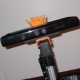ROS Resources: Documentation | Support | Discussion Forum | Index | Service Status | Q&A answers.ros.org

# While loop for Ros Python Rosserial [closed]

What can i do to avoid Ros from losing connection to arduino when using a while loop?

i have tried many methods and nothing worked.

When the program stays long in the turnToNorth position the rosserial connection is lost

Example of snippet code below:

#!/usr/bin/env python
import rospy
import time
from std_msgs.msg import String
from std_msgs.msg import Int32
from std_msgs.msg import Bool
from sensor_msgs.msg import Range
from marval.msg import l298n as l298n
from marval.msg import lsm303 as lsm303

subMotor = l298n()
subServo = String()
subStart = Bool()
motorData = l298n()
subCompass = lsm303()

motorSpeed = 23
irEdgeDistance = 1.0
usDistance = 0.15
moveAngle = 20.0
intervalTime = 0
startDirection = 0.0

def subStartC(data):
global subStart
subStart = data

def subCompassC(data):
global subCompass
subCompass = data

#Publishers
pubMotor = rospy.Publisher('motor_data', l298n, queue_size=10)

#Subscribers
rospy.Subscriber('start_data', Bool, subStartC)
rospy.Subscriber('compass_data', lsm303, subCompassC)

def moveRobot():
turnToNorth()

def turnToNorth():
global startDirection
while (int(subCompass.angle) != int(startDirection) and not rospy.is_shutdown()):
motorData.enA = motorSpeed
motorData.enB = motorSpeed
motorData.in1 = 0
motorData.in2 = 1
motorData.in3 = 1
motorData.in4 = 0
pubMotor.publish(motorData)

def startRobot():
#initiate node
rospy.init_node('moveRobot', anonymous=True)
rate = rospy.Rate(5) #hz
while not rospy.is_shutdown():
if(subStart.data == True):
moveRobot()  #starting the robot movement
else
stopRobot()
rate.sleep()

if __name__ == '__main__':

try:
startRobot()

except rospy.ROSInterruptException:
pass


Part 2 :(EDIT)

def turnToNorth():
global startDirection
while True:
if(int(subCompass.angle) == int(startDirection)):
break
else:
motorData.enA = motorSpeed
motorData.enB = motorSpeed
motorData.in1 = 0
motorData.in2 = 1
motorData.in3 = 1
motorData.in4 = 0
pubMotor.publish(motorData)

edit retag reopen merge delete

### Closed for the following reason the question is answered, right answer was accepted by RosStudent close date 2018-04-01 02:20:27.283004

i have tried many methods and nothing worked

What have you tried and what were the results?

When in the loop after some seconds rosserial disconnects from arduino and the program keepa looping and cannot terminate the program.

I also tried while True: and add a break when condition is met but still same issue.

Can you please update your question with a copy and paste of the code that you've tried and the results?

Sort by » oldest newest most votedThere may be other issues, but the thing that jumps out to me is this:

In your function "turnToNorth()" you have a publish call in a while() loop, with no sleeps. This is probably running at 10khz+, and overflowing some buffer somewhere (you can't possibly shove that much data down a serial connection). At least put a rospy.rate() or time.sleep() in that loop, so that it runs no more than maybe 25hz to start with.

more

I'll will do that as I have other functions similar to the turnToNorth. Furthermore what happens if it stays for around 10sec in a while loop? Should there be an issue?

Yes creating the below with each publisher in a while loop fixed the issue.

r = rospy.rate(5)

while True:
pub.publish(data)
r.sleep()
`
more